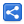# Precision and Scale of a Number

[Last Updated: Mar 7, 2017]

Precision is the total number of significant digits in a number.

Scale is the number of digits to the right of the decimal point.

### Examples:

Number Precision Scale
123.456 6 3
10 2 0
-96.9923 6 4
0.0 1 1

### Negative Scale

For a negative scale value, we apply the following formula:

result = (given number) * 10 -(scale value)

### Example

```Given number = 1234.56
scale = -5

->  (1234.56) * 10-(-5)
->  (1234.56) * 10+5
->  123456000
```

 Share